Courses
Courses for Kids
Free study material
Offline Centres
More

# NCERT Solutions for Class 10 Maths Chapter 9 - Some Applications Of TrigonometryLast updated date: 01st Dec 2023
Total views: 777.3k
Views today: 8.77k

## NCERT Solutions for Class 10 Maths Chapter 9 Some Applications of Trigonometry - Free PDF

Chapter 9 of the class 10 maths syllabus is on Applications of Trigonometry. It is an important chapter that is covered in class 10 and is divided into 3 major sections. We recommend that students take time to read through these topics very carefully so that they do not miss out on any important information that is provided in our solutions. Our expert teachers have invested their time in curating these exam-appropriate solutions to best suit the needs of the students to aid them in scoring well in their examinations.

The following table has been provided to give the students a glimpse at the subtopics under the chapter on Applications of Trigonometry.

 Sl. No. Important Topics 1. Introduction 2. Heights and Distances 3. Summary

### Importance of Applications of Trigonometry

The branch of mathematics that deals with relations of the sides and angles of triangles and the related functions of angles of triangles is known as Trigonometry.

The application of trigonometry is an important area that students in class 10 should concentrate on. These applications will not just help in solving sums based on trigonometry in exams but will also aid in real-world calculations and measurements.

The NCERT Solutions for Class 10 Maths Chapter 9 Some Applications of Trigonometry gives a detailed explanation of all the questions given in the NCERT textbook. NCERT Solutions help you to score high marks in 10th CBSE board exams as well as increase your confidence level as all the trigonometry related concepts are well-explained in a structured way. The Vedantu expert teachers have prepared the NCERT Solutions Class 10 Maths Chapter 9 some applications of trigonometry free pdfs as per the NCERT syllabus and guidelines given by the CBSE board. NCERT Solution is always beneficial in your exam preparation and revision. Download NCERT Solutions for Class 10 Maths from Vedantu, which are curated by master teachers. Also, you can revise and download Class 10 Science Solutions for Exam 2023-2024, using the updated CBSE textbook solutions provided by us.

### Key Features for NCERT Solutions for Class 10 Maths Chapter 9 Some Applications of Trigonometry

The key features of Class 10 Maths NCERT Solutions for Ch-9 Some Applications of Trigonometry are listed below.

• Provides a comprehensive explanation of the applications of trigonometry

• Easy to access on the Vedantu mobile app and website

• Step-by-step solutions for all sums

• Supporting explanations for self-study

• Solved by experts as per the updated CBSE syllabus and guidelines

Watch videos on
NCERT Solutions for Class 10 Maths Chapter 9 - Some Applications Of Trigonometry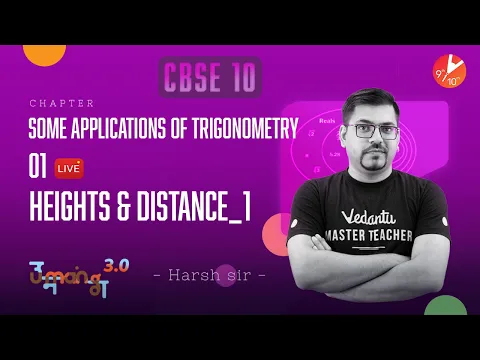Some Applications of Trigonometry L-1[ Heights & Distance Part-1] CBSE Class10 Maths Term2 | Vedantu
Vedantu 9&10
SubscribeShare
5.2K likes
122.6K Views
1 year ago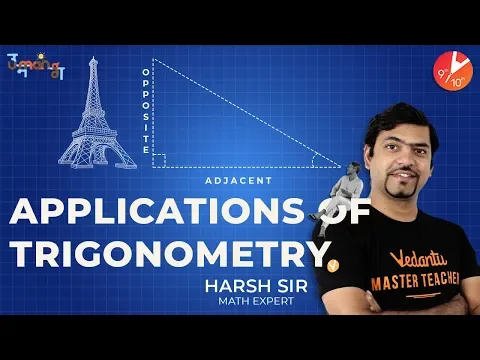Applications of Trigonometry | CBSE Class 10 Maths | NCERT Solutions | Vedantu Class 10
Vedantu 9&10
6.3K likes
170.2K Views
4 years ago

### Related Chapters

More Free Study Material for Some Applications of TrigonometryRevision notesImportant questionsNcert books

## Exercises under NCERT Solutions for Class 10 Maths Chapter 9 Some Applications of Trigonometry

Exercise 9.1: This exercise covers four questions related to finding angles of elevation and depression. The questions involve finding the height, distance, or both of an object using trigonometric ratios. The solutions provided aim to help students understand the practical application of trigonometry in real-life situations.

## Access NCERT Solutions for Class 10 Maths Chapter 9 - Some Applications Of Trigonometry

Exercise- 9.1

1. A circus artist is climbing a $20m$ long rope, which is tightly stretched and tied from the top of a vertical pole to the ground. Find the height of the pole, if the angle made by the rope with the ground level is $\mathbf{30}{}^\circ$.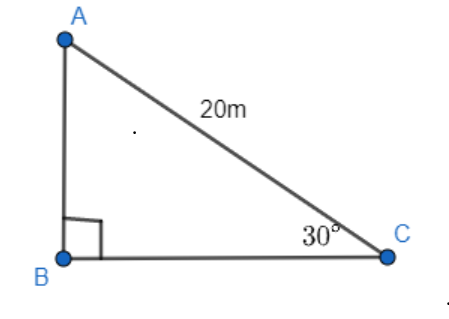Ans: By observing the figure, $AB$ is the pole.

In$\Delta ABC$,

$\frac{\text{AB}}{\text{AC}}=\sin {{30}^{{}^\circ }}$

$\Rightarrow \frac{\text{AB}}{20}=\frac{1}{2}$

$\Rightarrow \text{AB}=\frac{20}{2}=10$

Therefore, the height of the pole is$10m$.

2. A tree breaks due to storm and the broken part bends so that the top of the tree touches the ground making an angle $\mathbf{30}{}^\circ$with it. The distance between the foot of the tree to the point where the top touches the ground is $\mathbf{8m}$. Find the height of the tree.

Ans: Let $AC$was the original tree. Due to the storm, it was broken into two parts. The broken part  $AB$ is making 30° with the ground.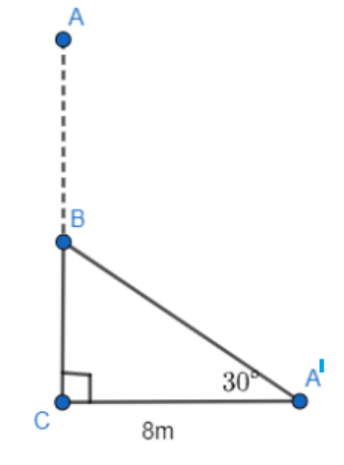Let $\mathrm{AC}$ be the original tree. Due to the storm, it was broken into two parts. The broken part $\mathrm{A}^{\prime} \mathrm{B}$ is making ${{30}^{{}^\circ }}$ with the ground. In triangle${{\text{A}}^{\prime }}\text{BC}$,

$\Rightarrow \frac{BC}{{{A}^{\prime }}C}=\tan {{30}^{{}^\circ }}$

$\Rightarrow \frac{BC}{8}=\frac{1}{\sqrt{3}}$

$\Rightarrow \text{BC}=\left( \frac{8}{\sqrt{3}} \right)\text{m}$

$\Rightarrow \frac{{{\text{A}}^{\prime }}\text{C}}{{{\text{A}}^{\prime }}\text{B}}=\cos 30$

$\Rightarrow \frac{8}{{{A}^{\prime }}B}=\frac{\sqrt{3}}{2}$

$\Rightarrow {{A}^{\prime }}B=\left( \frac{16}{\sqrt{3}} \right)m$

Height of tree $=\mathrm{A}^{\prime} \mathrm{B}+\mathrm{BC}$

$=\left(\frac{16}{\sqrt{3}}+\frac{8}{\sqrt{3}}\right) \mathrm{m}=\frac{24}{\sqrt{3}} \mathrm{~m}$

$=8 \sqrt{3} \mathrm{~m}$

3. A contractor plans to install two slides for the children to play in a park. For the children below the age of $5$ years, she prefers to have a slide whose top is at a height of$\mathbf{1}.\mathbf{5m}$, and is inclined at an angle of $\mathbf{30}{}^\circ$ to the ground, whereas for the elder children she wants to have a steep slide at a height of$\mathbf{3m}$, and inclined at an angle of $60{}^\circ$ to the ground. What should be the length of the slide in each case?

Ans: It can be observed that $\text{AC}$ and $\text{PR}$ are the slides for younger and elder children respectively.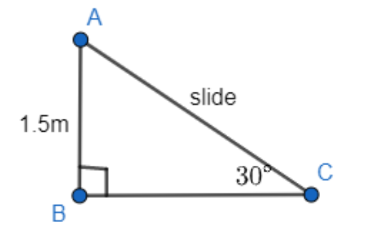In $\vartriangle \text{ABC}$

$\Rightarrow \frac{\text{AB}}{\text{AC}}=\sin 30$

$\Rightarrow \frac{1.5}{\text{AC}}=\frac{1}{2}$

$\Rightarrow \text{AC}=3~\text{m}$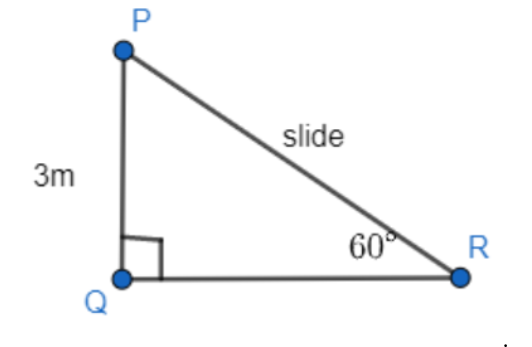In $\vartriangle \text{PQR}$,

$\Rightarrow \frac{\text{PQ}}{\text{PR}}=\sin {{60}^{{}^\circ }}$

$\Rightarrow \frac{3}{\text{PR}}=\frac{\sqrt{3}}{2}$

$\Rightarrow \text{PR}=\frac{6}{\sqrt{3}}=2\sqrt{3}~\text{m}$

Therefore, the lengths of these slides are $3~\text{m}$ and $2\sqrt{3}~\text{m}$.

4. The angle of elevation of the top of a tower from a point on the ground, which is$\mathbf{30m}$ away from the foot of the tower is $\mathbf{30}{}^\circ .$ Find the height of the tower.

Ans: Let $\mathrm{AB}$ be the tower and the angle of elevation from point $\mathrm{C}$ (on ground) is $30^{\circ}$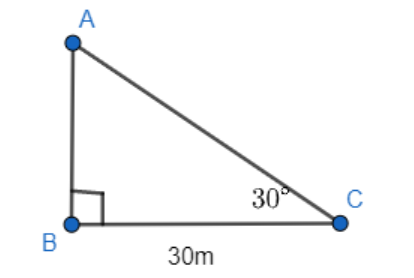In $\vartriangle \text{ABC}$ ,

$\Rightarrow \frac{\text{AB}}{\text{BC}}=\tan {{30}^{{}^\circ }}$

$\Rightarrow \frac{\text{AB}}{30}=\frac{1}{\sqrt{3}}$

$\Rightarrow \text{AB}=\frac{30}{\sqrt{3}}=10\sqrt{3}~\text{m}$

Therefore, the height of the tower is $10\sqrt{3}~\text{m}$.

5. A kite is flying at a height of $\mathbf{60m}$ above the ground. The string attached to the kite is temporarily tied to a point on the ground. The inclination of the string with the ground is $\mathbf{60}{}^\circ .$ Find the length of the string, assuming that there is no slack in the string.

Ans: Let $\text{K}$ be the kite and the string is tied to point $\text{P}$ on the ground.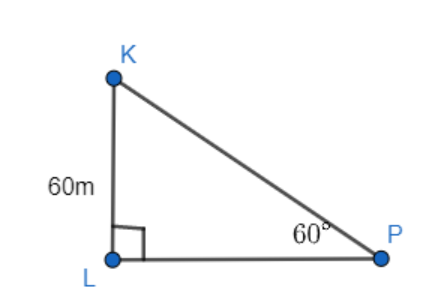In $\vartriangle \text{KLP}$,

$\Rightarrow \frac{\text{KL}}{\text{KP}}=\sin {{60}^{{}^\circ }}$

$\Rightarrow \frac{60}{\text{KP}}=\frac{\sqrt{3}}{2}$

$\Rightarrow \text{KP}=\frac{120}{\sqrt{3}}=40\sqrt{3}~\text{m}$

Hence, the length of the string is $40\sqrt{3}~\text{m}$.

6. A $\mathbf{1}.\mathbf{5m}$ tall boy is standing at some distance from a $\mathbf{30m}$ tall building. The angle of elevation from his eyes to the top of the building increases from $\mathbf{30}{}^\circ$ to $\mathbf{60}{}^\circ$ as he walks towards the building. Find the distance he walked towards the building.

Ans : Let the boy was standing at point S initially. He walked towards the building and reached at point T.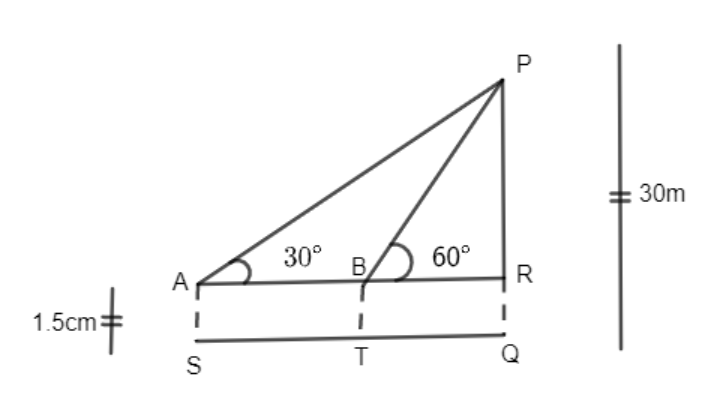$\text{PR}=\text{PQ}-\text{RQ}$

$=(30-1.5)=28.5~=\frac{57}{2}~$

In $\vartriangle \text{PAR}$,

$\Rightarrow \frac{\text{PR}}{\text{AR}}=\tan {{30}^{{}^\circ }}$

$\Rightarrow \frac{57}{2\text{AR}}=\frac{1}{\sqrt{3}}$

$\Rightarrow \text{AR}=\left( \frac{57}{2}\sqrt{3} \right)\text{m}$

In $\vartriangle \text{PRB}$,

$\Rightarrow \frac{\text{PR}}{\text{BR}}=\tan {{60}^{{}^\circ }}$

$\Rightarrow \frac{57}{2B\text{R}}=\sqrt{3}$

$\Rightarrow \text{BR}=\frac{57}{2\sqrt{3}}\text{=}\frac{19\sqrt{3}}{2}m$

By observing the figure,

$ST=AB$

$=AR-BR=\left( \frac{57\sqrt{3}}{2}-\frac{19\sqrt{3}}{2} \right)$

$=\left( \frac{38\sqrt{3}}{2} \right)=19\sqrt{3}m$

Hence, he walked $19\sqrt{3}m$ towards the building.

7. From a point on the ground, the angles of elevation of the bottom and the top of a transmission tower fixed at the top of a $\mathbf{20m}$ high building are $\mathbf{45}{}^\circ$ and $\mathbf{60}{}^\circ$ respectively. Find the height of the tower.

Ans: Let $AB$ be the statue, $BC$ be the pedestal, and $D$ be the point on the ground from where the elevation angles are to be measured.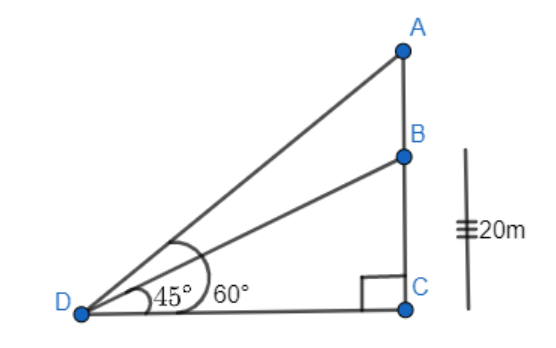In $\Delta \text{BCD}$,

$\Rightarrow \frac{\text{BC}}{\text{CD}}=\tan {{45}^{{}^\circ }}$

$\Rightarrow \frac{\text{BC}}{\text{CD}}=1$

$\Rightarrow \text{BC}=\text{CD}$

In $\Delta A\text{CD}$,

$\Rightarrow \frac{\text{AB}+\text{BC}}{\text{CD}}=\tan {{60}^{{}^\circ }}$

$\Rightarrow \frac{\text{AB}+\text{BC}}{\text{CD}}=\sqrt{3}$

$\frac{AB+20}{20}=\sqrt{3}$

$AB=\left( 20\sqrt{3}-20 \right)m$

$=20\left( \sqrt{3}-1 \right)m$

8.  A statue, $\mathbf{1}.\mathbf{6m}$ tall, stands on a top of pedestal, from a point on the ground, the angle of  elevation of the top of statue is $\mathbf{60}{}^\circ$ and from the same point the angle of elevation of the  top of the pedestal is $\mathbf{45}{}^\circ .$ Find the height of the pedestal.

Ans: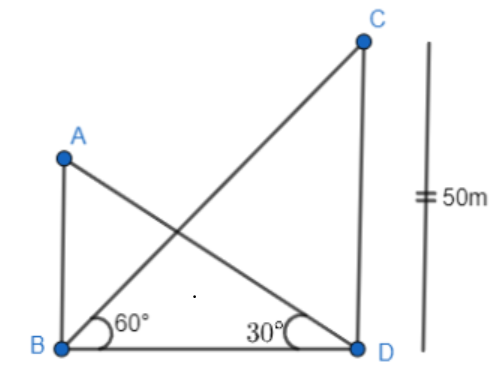Let AB be the statue, BC be the pedestal, and D be the point on the ground from where  the elevation angles are to be measured.

$\text{ In }\vartriangle \text{BCD,}$

$\text{ }\frac{\text{BC}}{\text{CD}}=\tan 45$

$\frac{\text{BC}}{\text{CD}}=1$

$\text{BC}=\text{CD }$

$\text{In }\vartriangle \text{ACD, }$

$\frac{\text{AB}+\text{BC}}{\text{CD}}=\tan {{60}^{{}^\circ }}$

$\frac{\text{AB}+\text{BC}}{\text{CD}}=\sqrt{3}\text{ }$

$1.6+\text{BC}=\text{BC}\sqrt{3}\quad [\text{As}\,\,\text{CD}=\text{BC}]\,\,$

$\text{BC}(\sqrt{3}-1)=1.6\,\,\,$

$\text{BC}=\frac{(1.6)(\sqrt{3}+1)}{(\sqrt{3}-1)(\sqrt{3}+1)}\quad [\text{ByRationalization}]$

$=\frac{1.6(\sqrt{3}+1)}{{{(\sqrt{3})}^{2}}-{{(1)}^{2}}}$

$=\frac{1.6\left( \sqrt{3}+1 \right)}{2}=0.8\left( \sqrt{3}+1 \right)m$

9. The angle of elevation of the top of a building from the foot of the tower is $\mathbf{30}{}^\circ$ and the angle of elevation of the top of the tower from the foot of the building is $\mathbf{60}{}^\circ .$ If the tower is $\mathbf{50m}$ high, find the height of the building.

Ans: Let $AB$be the building and $CD$be the tower.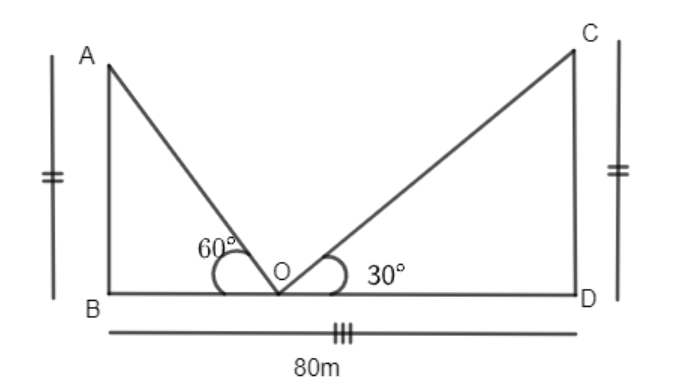In $\Delta \text{CDB}$,

$\Rightarrow \frac{\text{CD}}{\text{BD}}=\tan {{60}^{{}^\circ }}$

$\Rightarrow \frac{50}{\text{BD}}=\sqrt{3}$

$\Rightarrow \text{BD}=\frac{50}{\sqrt{3}}$

In $\Delta ABD$

$\Rightarrow \frac{AB}{BD}=\tan 30{}^\circ$

$\Rightarrow AB=\frac{50}{\sqrt{3}}\left( \frac{1}{\sqrt{3}} \right)=\frac{50}{3}=16\frac{2}{3}$

Therefore, the height of the building is $16\frac{2}{3}$m.

10. Two poles of equal heights are standing opposite each other on either side of the road, which is $\mathbf{80m}$wide. From a point between them on the road, the angles of elevation of the top of the poles are $\mathbf{60}{}^\circ$and $\mathbf{30}{}^\circ$respectively. Find the height of poles and the distance of the point from the poles.

Ans: Let $AB$ and $CD$ be the poles and $O$ is the point from where the elevation angles are measured.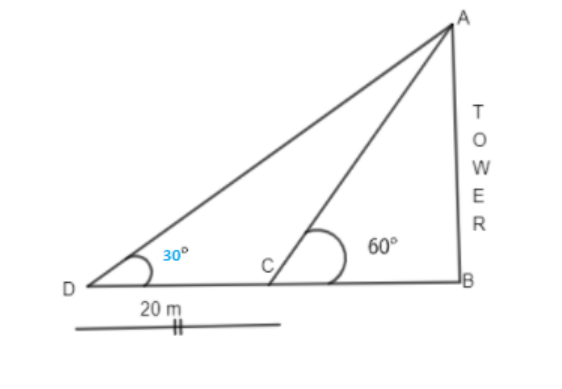In $\Delta \mathrm{CDO}$,

$\Rightarrow \frac{\text{AB}}{\text{BO}}=\tan {{60}^{{}^\circ }}$

$\Rightarrow \frac{\text{AB}}{\text{BO}}=\sqrt{3}$

$\Rightarrow \text{BO}=\frac{\text{AB}}{\sqrt{3}}$

In $\Delta \text{CDO}$,

$\Rightarrow \frac{\text{CD}}{\text{DO}}=\tan {{30}^{{}^\circ }}$

$\Rightarrow \frac{\text{CD}}{80-\text{BO}}=\frac{1}{\sqrt{3}}$

$\Rightarrow \text{CD}\sqrt{3}=80-\text{BO}$

$\Rightarrow \text{CD}\sqrt{3}=80-\frac{\text{AB}}{\sqrt{3}}$

$\Rightarrow \text{CD}\sqrt{3}+\frac{\text{AB}}{\sqrt{3}}=80$

Since the poles are of equal heights, $\text{CD}=\text{AB}$

$\Rightarrow \text{CD}\left[ \sqrt{3}+\frac{1}{\sqrt{3}} \right]=80$

$\Rightarrow \text{CD}\left( \frac{3+1}{\sqrt{3}} \right)=80$

$\Rightarrow \text{CD}=20\sqrt{3}~\text{m}$

By observing the figure,

$\Rightarrow BO=\frac{AB}{\sqrt{3}}=\frac{CD}{\sqrt{3}}=\frac{20\sqrt{3}}{\sqrt{3}}=20m$

$\Rightarrow DO=BD-BO=80-20=60m$

Therefore, the height of poles is $20\sqrt{3}$ and the point is $20m$ and $60m$ far from these poles.

11. A TV tower stands vertically on a bank of a canal. From a point on the other bank directly opposite the tower the angle of elevation of the top of the tower is $\mathbf{60}{}^\circ .$ From another point $\mathbf{20m}$away from this point on the line joining this point to the foot of the tower, the angle of elevation of the top of the tower is $\mathbf{30}{}^\circ .$ Find the height of the tower and the width of the canal.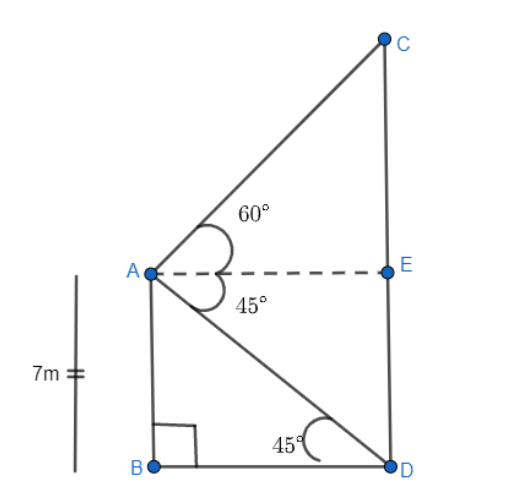Ans: In $\Delta \text{ABC}$,

$\Rightarrow \dfrac{\text{AB}}{\text{BC}}=\tan {{60}^{{}^\circ }}$

$\Rightarrow \dfrac{\text{AB}}{\text{BO}}=\sqrt{3}$

$\Rightarrow \text{BC}=\frac{\text{AB}}{\sqrt{3}}$…. (1)

In $\Delta ABD$,

$\Rightarrow \dfrac{AB}{\text{BD}}=\tan {{30}^{{}^\circ }}$

$\Rightarrow \dfrac{AB}{BC+CD}=\frac{1}{\sqrt{3}}$

$\Rightarrow \dfrac{AB}{\frac{AB}{\sqrt{3}}+20}=\dfrac{1}{\sqrt{3}}$

$\Rightarrow \dfrac{AB\sqrt{3}}{AB+20\sqrt{3}}=\dfrac{1}{\sqrt{3}}$

$\Rightarrow 3AB=AB+20\sqrt{3}$

$\Rightarrow 2AB=20\sqrt{3}$

$\Rightarrow AB=10\sqrt{3}m$

Substitute $AB=10\sqrt{3}m$ in $\text{BC}=\dfrac{\text{AB}}{\sqrt{3}}$,

$\Rightarrow BC=\dfrac{AB}{\sqrt{3}}=\dfrac{10\sqrt{3}}{\sqrt{3}}=10m$

Therefore, the height of the tower is $10\sqrt{3}m$ and the width of the canal is $10m$.

12. From the top of a $\mathbf{7m}$ high building, the angle of elevation of the top of a cable tower is $\mathbf{60}{}^\circ$ and the angle of depression of its foot is $\mathbf{45}{}^\circ .$ Determine the height of the tower.

Ans: Let $AB$ be a building and $CD$ be a cable tower.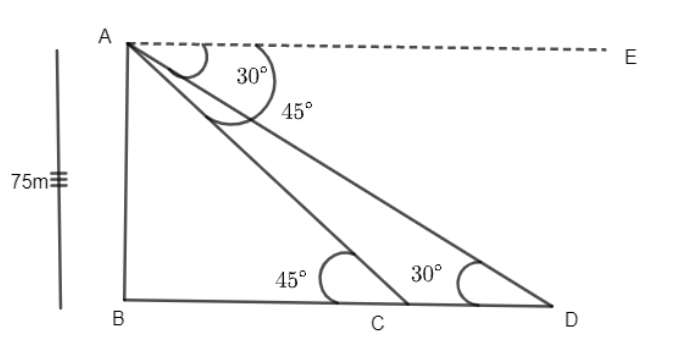In $\Delta \text{ABD}$,

$\Rightarrow \frac{\text{AB}}{\text{BD}}=\tan {{45}^{{}^\circ }}$

$\Rightarrow \frac{7}{\text{BD}}=1$

$\Rightarrow \text{BD=7m}$

In $\Delta \text{ACE}$, $AE=BD=7m$

$\Rightarrow \frac{\text{CE}}{AE}=\tan {{60}^{{}^\circ }}$

$\Rightarrow \frac{\text{CE}}{7}=\sqrt{3}$

$\Rightarrow \text{CE=7}\sqrt{3}\text{m}$

$\Rightarrow CD=CE+ED=\left( 7\sqrt{3}+7 \right)=7\left( \sqrt{3}+1 \right)m$

Therefore, the height of the cable tower is $7\left( \sqrt{3}+1 \right)m$.

13. As observed from the top of a $\mathbf{75m}$high lighthouse from the sea-level, the angles of depression of two ships are $\mathbf{30}{}^\circ$and $\mathbf{45}{}^\circ .$If one ship is exactly behind the other on the same side of the lighthouse, find the distance between the two ships.

Ans: Let $AB$be the lighthouse and the two ships be at point $C$ and $D$respectively.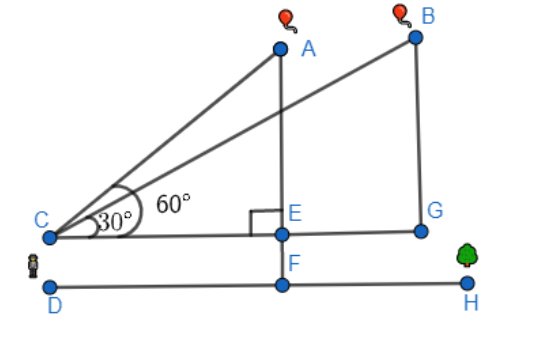In $\Delta \text{ABC}$,

$\Rightarrow \frac{\text{AB}}{\text{BC}}=\tan {{45}^{{}^\circ }}$

$\Rightarrow \frac{75}{\text{BC}}=1$

$\Rightarrow \text{BC=75m}$

In $\Delta \text{ABD}$,

$\Rightarrow \frac{AB}{BD}=\tan {{30}^{{}^\circ }}$

$\Rightarrow \frac{75}{BC+CD}=\frac{1}{\sqrt{3}}$

$\Rightarrow \frac{75}{75+CD}=\frac{1}{\sqrt{3}}$

$\Rightarrow 75\sqrt{3}=75+CD$

$\Rightarrow 75\left( \sqrt{3}-1 \right)m=CD$

Therefore, the distance between the two ships is $75\left( \sqrt{3}-1 \right)m.$

14. A $\mathbf{1}.\mathbf{2m}$ tall girl spots a balloon moving with the wind in a horizontal line at a height of $\mathbf{88}.\mathbf{2m}$ from the ground. The angle of elevation of the balloon from the eyes of the girl at any instant is $\mathbf{60}{}^\circ .$ After some time, the angle of elevation reduces to $\mathbf{30}{}^\circ .$ Find the distance travelled by the balloon during the interval.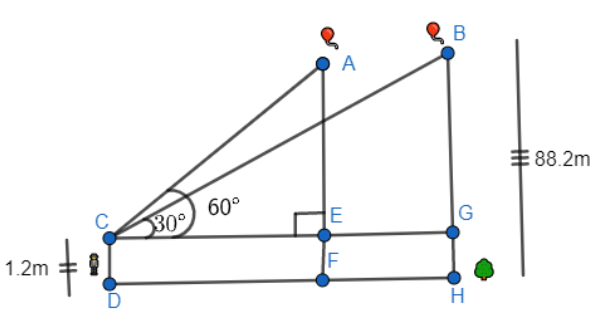Ans: Let the initial position $A$ of balloon change to $B$ after some time and $CD$ be the girl.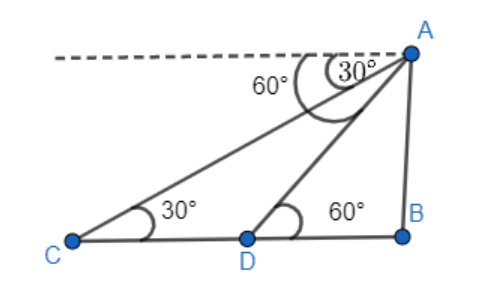In $\Delta \text{ACE}$,

$\Rightarrow \frac{\text{AE}}{\text{CE}}=\tan {{60}^{{}^\circ }}$

$\Rightarrow \frac{\text{AF-EF}}{\text{CE}}=\tan {{60}^{{}^\circ }}$

$\Rightarrow \frac{\text{88}\text{.2-1}\text{.2}}{\text{CE}}=\sqrt{3}$

$\Rightarrow \frac{\text{87}}{\text{CE}}=\sqrt{3}$

$\Rightarrow CE=\frac{87}{\sqrt{3}}=29\sqrt{3}m$

In $\Delta B\text{CG}$,

$\Rightarrow \frac{\text{BG}}{\text{CG}}=\tan {{30}^{{}^\circ }}$

$\Rightarrow \frac{\text{BH-GH}}{\text{CG}}=\frac{1}{\sqrt{3}}$

$\Rightarrow \frac{\text{88}\text{.2-1}\text{.2}}{\text{CG}}=\frac{1}{\sqrt{3}}$

$\Rightarrow \text{87}\sqrt{3}=CG$

Distance travelled by balloon$=EG=CG-CE$

$=\left( 87\sqrt{3}-29\sqrt{3} \right)$

$=58\sqrt{3}m$

15. A straight highway leads to the foot of a tower. A man standing at the top of the tower observes a car at an angle of depression of $\mathbf{30}{}^\circ ,$ which is approaching the foot of the tower with a uniform speed. Six seconds later, the angle of depression of the car is found to be $\mathbf{60}{}^\circ .$ Find the time taken by the car to reach the foot of the tower from this point.

Ans: Let $AB$ be the tower. Initial position of the car is $C$, which changes to $D$ after six seconds.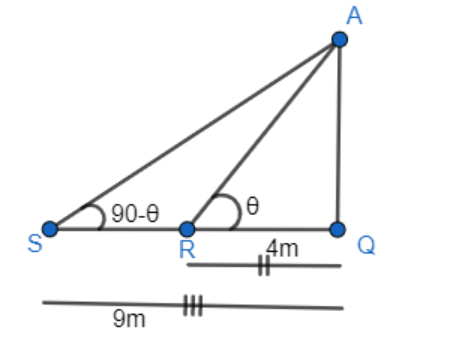In $\Delta \text{ADB}$,

$\Rightarrow \frac{\text{AB}}{CB}=\tan {{60}^{{}^\circ }}$

$\Rightarrow \frac{\text{AB}}{DB}=\sqrt{3}$

$\Rightarrow DB=\frac{AB}{\sqrt{3}}$

In $\Delta \text{ABC}$,

$\Rightarrow \frac{\text{AB}}{BC}=\tan {{30}^{{}^\circ }}$

$\Rightarrow \frac{\text{AB}}{BD+DC}=\frac{1}{\sqrt{3}}$

$\Rightarrow AB\sqrt{3}=BD+DC$

$\Rightarrow AB\sqrt{3}=\frac{AB}{\sqrt{3}}+DC$

$\Rightarrow DC=AB\sqrt{3}-\frac{AB}{\sqrt{3}}=AB\left( \sqrt{3}-\frac{1}{\sqrt{3}} \right)=\frac{2AB}{\sqrt{3}}$

Time taken by the car to travel distance DC $\left( i.e\frac{2AB}{\sqrt{3}} \right)=6$ seconds.

Time taken by the car to travel distance DB $\left( ie.,\frac{AB}{\sqrt{3}} \right)=\frac{6}{\frac{2AB}{\sqrt{3}}}\left( \frac{AB}{\sqrt{3}} \right)=\frac{6}{2}=3$ seconds.

16. The angles of elevation of the top of a tower from two points at a distance of $4m$and $9m$from the base of the tower and in the same straight line with it are complementary. Prove that the height of the tower is$6m$.

Ans: Let $AQ$be the tower and $R,S$are the points $4m,\text{ }9m$away from the base of the tower respectively. The angles are complementary. Therefore, if one angle is$\theta$, the other will be $90\text{ }-\text{ }\theta$

In $\Delta \text{AQR}$,

$\Rightarrow \frac{\text{AQ}}{QR}=\tan \theta$

$\Rightarrow \frac{\text{AQ}}{4}=\tan \theta$ …. (i)

In $\Delta \text{AQS}$,

$\Rightarrow \frac{\text{AQ}}{SQ}=\tan (90-\theta )$

$\Rightarrow \frac{AQ}{9}=\cot \theta$ ….. (ii)

On multiplying equations (i) and (ii), we obtain

$\Rightarrow \left( \frac{AQ}{4} \right)\left( \frac{AQ}{9} \right)=\left( \tan \theta \right)\left( \cot \theta \right)$

$\Rightarrow \frac{A{{Q}^{2}}}{36}=1$

$\Rightarrow A{{Q}^{2}}=36$

$\Rightarrow AQ=\sqrt{36}=\pm 6$

However, height cannot be negative.

Therefore, the height of the tower is $6m$.

## NCERT Solutions for Class 10 Maths Chapter 9 Some Applications of Trigonometry - PDF Download

You can opt for Chapter 9 - Some Applications of Trigonometry NCERT Solutions for Class 10 Maths PDF for Upcoming Exams and also You can Find the Solutions of All the Maths Chapters below.

NCERT Solutions for Class 10 Maths

In the Class 10 Maths Chapter 9, you will study about different ways in which trigonometry is used to find the height and distance of different objects without actually measuring them. This chapter is divided into 3 sections and one exercise. The first section is the basic introduction of trigonometry in which you will learn, how the need for the trigonometry arose and its application in different fields. The second section includes an introduction to height and distance, important terms related to the height and distance, conditions where the trigonometry concepts are used along with the examples, and last but not the least one exercise at the end. The questions asked in the exercise are based on the basic concepts of trigonometry and its application. The third section includes a summary of the chapter where some important terms given in the chapter are discussed.

### Some Applications of Trigonometry

List of topics and exercise covered in Class 10 Chapter 9 Some applications of trigonometry.

Section 9.1: Introduction to some applications of trigonometry

Section 9.2: Height and Distance

Exercise 9.1: Questions related to some applications of trigonometry. This exercise included 16 questions.

Section 9.3: Summary of the chapter

In the ch 9 Maths class 10, we will be learning about trigonometry, some application of trigonometry, and the entire summary of the chapter.

### Overview of the Exercises Covered in the NCERT Solutions Class 10 Maths Ch-9 Some Applications of Trigonometry

The sums of Chapter 9 Class 10 Maths are based on the concepts of trigonometry and their applications. Students are advised to learn and revise all the important formulas and theories of trigonometry to have a better grasp of these NCERT Solutions. The sums mostly involve computing various heights and distances using trigonometric ratios. Students should revise the values of some trigonometric ratios like sin, cos, tan, cot, cosec, and sec for the commonly used measures of theta.

Hence, by practising and referring to the NCERT Solutions for Class 10 Maths Chapter 9 Some Applications of Trigonometry, students will get an overview of how the trigonometric ratios are employed in day-to-day real-world scenarios. For example, the sums that involve using the trigonometric ratios to calculate the heights and angles for poles, buildings, towers, etc., are going to provide them with this kind of knowledge.

### What is Trigonometry?

Trigonometry is one of the most historical subjects studied by different scholars throughout the world. As you have read in Chapter 8 that trigonometry was introduced because its requirement arose to astronomy. Since then trigonometry is used to calculate the distance from the Earth to the stars and the planets. The most important use of trigonometry is to find out the height of the highest mountain in the world i.e. Mount Everest which is named after Sir George Everest. It is also widely used in Geography and navigation. The knowledge of trigonometry enables us to construct maps, evaluate the position of an island concerning the longitudes and latitudes.

### Historical Facts. Let us Turn to the History of Trigonometry

The trigonometry was used by surveyors for centuries. One of the notable and the largest surveying projects of the nineteenth century was the “ Great Trigonometric Survey" of British India for which the two largest theodolites were constructed. The highest mountain in the world was discovered during the survey in 1852. From a distance of over 160 km, this peak was seen from 6 distinct stations. This peak was named after Sir George Everest who had first used the theodolites. Theodolites are now exhibited in the museum of the surveys of Dehradun.

### Theodolites

Surveying instrument, which is used for measuring angles with a rotating telescope

### Height and Distance

In this topic, you will study about the line of sight, angle of elevation, horizontal level, and angle of depression. All these terms are explained in a detailed form along with some solved examples based on it. These solved examples based on the terms line of sight, angle of elevation and angle of depression will help you to understand the concepts thoroughly.

### How to Calculate Height and Distance?

Trigonometric ratios are used to find out the height and the distance of the object. For example: In figure 1, you can see a boy looking at the top of the lampost. AB is considered as the horizontal level. This level is stated as the line parallel to the ground passing through the viewer's eyes. AC is considered as the line of sight. ∠A is known as the angle of elevation. Similarly, in figure 2, you can see PQ is the line of sight, PR is the horizontal level and ∠P is known as the angle of elevation.

An inclinometer or Clinometer is a device usually used for measuring the angle of elevation and the angle of depression.

Let us recall some trigonometric ratios which help to solve the questions based on  class 10 maths Chapter 9.

### Trigonometry Ratios

The ratio of the sides of a right-angle triangle in terms of any of its acute angle triangle is known as the trigonometric ratio of that specific angle.

In terms of ∠C, the ratio of trigonometry are given as:

Sine - The sine of an angle is stated as the ratio of the opposite side ( perpendicular side) to that angle to the hypotenuse side.

Hence, Sine C = Opposite side/Hypotenuse side

Cosine- The cosine of an angle is stated as the ratio of the adjacent side to that angle to the hypotenuse side.

Hence, Cosine C = Adjacent side /Hypotenuse side

Tangent - The tan of an angle is stated as the ratio of the opposite side (perpendicular side) to that angle to the side adjacent to that angle.

Hence, Tan C = Opposite side/Adjacent side

Cosecant- It is the reciprocal of sine.

Hence, Cosec C = Hypotenuse side/Opposite side

Secant- It is the reciprocal of cosine.

Hence, Sec C= Hypotenuse side/Adjacent side

Cotangent- It is the reciprocal of tangent.

Hence, Cot C = Adjacent side/Opposite side

The following trigonometry ratio table is used to calculate the questions based on applications of trigonometry class 10 NCERT solutions.

### Trigonometric Ratio Table

 ∠C 0° 35° 45° 60° 90° Sin C 0 1/2 1/2 3/2 1 Cos C 1 3/2 1/2 1/2 0 Tan C 0 1/3 1 3 Not defined Cosec C Not defined 2 2 2/3 1 Sec C 1 2 / 3 2 2 Not defined Cot C Not defined 3 1 1/3 0

Now, you must have understood all the important topics and terms covered in each section of class 10 maths chapter 9. Perfect understanding of NCERT class 10 chapter 9 Introduction helps you to focus on some points such as the weightage of the chapter, important questions that can be asked in the examination, types of questions that can be appeared in your, etc. This will help you to solve the exam more confidently and also ensures you that you can finish your exam within a time-duration.

As, there is a proverb that says "Practice makes the men perfect". It tells us the importance of practicing continuously in any subject to learn anything. Continuous practice is a must to learn any of the subjects. Practicing class 10 maths Chapter 9 NCERT solutions designed by Vedantu experts will bring accuracy and confidence in you as they are designed according to the caliber of the students. It helps you to increase the speed of solving your problems and also bring more accuracy in you. With practicing NCERT questions more and more, you will be aware of the types of questions that can be asked in the examination. This will help you to solve your exam paper more confidently. Practicing not merely enhances your conceptual understanding but also enhances your logical reasoning. Most of the time the questions asked in the examination are repeated  and solving the previous questions helps you to solve the questions speedily and accurately in the exam. By following the above-mentioned points, you can surely score above 90% in your board exam.

### Important features of the NCERT Solutions for Class 10 Maths Chapter 9- Some Applications of Trigonometry

• The solutions are designed by the subject experts of Vedantu.

• Chapter-wise questions and solutions are easily accessible.

• Special guidance for the students preparing for their board examinations.

• Exercise questions are easily accessible.

• The solutions are well-explained in the comprehensive method.

How Vedantu NCERT Solutions for class 10 maths Chapter 9 -Some applications of trigonometry will help you to score good marks in your board exams?

NCERT Solution for class 10 plays an important role in shaping the future of the students as the grades which the students will score will shape the future of the student. The NCERT solution prepared by the professionals of Vedantu is a one-stop solution for all your queries related to class 10 maths chapter 9. Detailed explanation and stepwise solutions for each question prepared by the experts will help you to understand the concept in a better way. The NCERT solutions prepared by the experts of Vedantu provides excellent material for the student to practice and make the learning process more effective.

The importance of the Vedantu’s NCERT Solutions for Class 10 Maths Chapter 9-Some applications of trigonometry are:

• Solutions are framed keeping in mind the age of the students.

• The content of the topic is pointed, brief, and straightforward.

• Complex questions are divided into small parts and well-explained to save the students from taking the unnecessary strain.

• Every question is explained with the relevant image to understand the question precisely.

• The solutions are designed under the latest syllabus and CBSE guidelines.

Vedantu experts tried their level best to provide your NCERT Solutions for Class 10 Maths Chapter 9. The aim to provide the solution is to help the students to solve each question given in the board exams in no time.

### Why are Some Applications of Trigonometry Important?

Class 10 Chapter 9 some application of trigonometry is an important topic to discuss as it tells how trigonometry is used to find the height and distance of different objects such as the height of the building, the distance between the Earth and Planet and Stars, the height of the highest mountain Mount Everest, etc.

To solve the questions based on some applications of trigonometry class 10, it is necessary to remember trigonometry formulas, trigonometric relations, and values of some trigonometric angles. The following are the concepts covered in the 'height and distance' Some applications of trigonometry.

• To measure the height of big towers or big mountains

• To determine the distance of the shore from the sea.

• To find out the distance between two celestial bodies.

This chapter has a weightage of 12 marks in class 10 Maths Cbse (board) exams.

One question can be expected from this chapter. Class 10 Maths CBSE paper is divided into 4 parts and each question comes with different marks. The questions will be allocated with 1 mark, 2 marks, 3 marks or 4 marks.

Discussion about the sections, exercise, and type of questions given in the exercise.

The important topic “ Height and Distance" covered in Some applications of trigonometry class 10 is followed by one exercise with 16 questions. The exercise aims to test your knowledge and how deeply you understood each formula and concept of the topic. The numerical questions given in this chapter are based on some applications of trigonometry.

To make you understand the topic and related concept, solved numerical problems are also given. Stepwise solutions are given for each of the solved examples. It will help you to understand which concept and formula will be used to solve the given questions accurately.

### Section 9.1 - Introduction

This section gives an introduction to some applications of trigonometry. It tells you how trigonometry is used by different scholars throughout the world and its uses in different fields.  It also tells you the way trigonometry is used to find the height and distance of different objects without actually measuring them.

### Section 9.2: Height and Distance

In this section, some important terms such as a line of sight, horizontal level, angle of elevation, and angle of depression are discussed. All these important terms are discussed along with the solved examples based on them which will clear your concepts thoroughly and also helps you to solve the questions given in the exercise.

### Exercise 9.1: “Height and Distance”

This exercise includes a total of 16 questions. Each question asked in the exercise are based on the concept of “Height and Distance”.

### Description of the Questions Asked in Exercise 9.1

 Question No. Given Information To Calculate 1. The angle of elevation and the length of the rope are given We have to calculate the height of the tower. 2. The distance of the object and angle of elevation are given We have to calculate the height of the tree 3. The angle of elevation and height of the two slides are given We need to calculate the length of the slide 4. The distance of the object and angle of elevation are given We have to calculate the height of the tower. 5. Height of the object and the distance of the object are given We have to calculate the height of the tower. 6. The angle of depression and height of the observer from the ground are given We have to calculate the distance between two objects 7. The angle of elevation from the ground to the bottom of the tower and angle of elevation from the ground to the top of the tower are given We have to calculate the height of the tower. 8. Length of the statue, angle of elevation to the top of the statue and angle of elevation to the top of the pedestal are given We have to calculate the height of the pedestal 9. The angle of elevation of the top of the building from the foot of the tower, Angle of elevation of the top of the tower from the foot of the building and height of the tower are given We have to calculate the height of the building 10. Angles of elevations of the top of the two towers and distance between the two poles are given We have to calculate the height of the tower and the distance of the point from the poles. 11. One angle of elevation from the bank of the river and another angle of elevation 20m away from the bank  of the river are given To calculate: Height of the tower, width of the canal 12. The angle of elevation, angle of depression and the length of the top of the building are given We have to calculate the height of the tower 13. The angle of depression of two ships and the height of lighthouse from the sea level is given We have to calculate the distance between two ships 14. The angle of elevation from one point to the top of the tower and angle of elevation from another point to the top of the tower are given We have to calculate the height of the tower and width of the canal 15. A man first observe the car at an angle of depression of 30°After 6 seconds, a man again observe the car at an angle of depression of 60°. We have to calculate the time taken by the car to reach the foot of the tower 16. Angles of elevation from one point and angle of elevation from another point are complementary and also the distance between two points from where the angle of elevation is formed is 4 m and 9 m. To prove: Height of the tower 6 m

### Section 9.3: Summary

The summary at the end of the chapter details a brief explanation of all the topics you covered in this chapter.

### Important Terms to Remember in Height and Distance

Line of Sight - It is a line that is drawn from the eye of an observer to the point on the object viewed by the observer.

The Angle of Elevation - It is defined as an angle that is formed between the horizontal line and line of sight. If the line of sight lies upward from the horizontal line, then the angle formed will be termed as an angle of elevation.

Let us take another situation when a boy is standing on the ground and he is looking at the object from the top of the building. The line joining the eye of the man with the top of the building is known as the line of sight and the angle drawn by the line of sight with the horizontal line is known as angle of elevation.

In the above figure line of sight is forming an angle θ through the horizontal line.This angle is known as the angle of elevation.

The Angle of Depression - It is defined as an angle drawn between the horizontal line and line of sight. If the line of sight lies downward from the horizontal line, then the angle formed will be termed as an angle of depression.

Let us take a situation when a boy is standing at some height concerning the object he is looking at. In this case, the line joining the eye of the man with the bottom of the building is known as the line of sight and the angle drawn by the line of sight with the horizontal line is known as angle of depression.

In the above figure angle, θ is considered as the angle of depression

Note: Angle of elevation is always equal to the angle of depression

### The important Point to Remember

The distance of the object is also considered as the base of the right angle triangle drawn through the height of the object and the line of sight.

The length of the horizontal level is also known as the distance of the object it forms the base of the triangle.

Line of sight is considered as a hypotenuse of the right-angle triangle. Hypotenuse side is calculated using Pythagorean Theorem if the height and distance of the object are given.

### Benefits of NCERT Solutions for Class 10 Maths

The benefits of the NCERT Solutions for Class 10 Maths Chapter 9 Some applications of Trigonometry are as follows.

• According to the guidelines set by CBSE, all answers are written.

• The content, which is short and self-explanatory, and well organized.

• To enhance the understanding of the topic, some of the answers include relevant infographics and images.

• During the revision of the test, it is useful and acts as a note.

• For you to solve the maximum questions and to get the chapter's command, solutions are kept simple.

• In chapter Linear Equations in two variables, these NCERT Solutions are written specifically so that students do not face any difficulties while solving any questions.

• Our experts have made sure that it is very easy to grasp these NCERT Solutions.

• In the form of solutions, it covers the whole syllabus and theory.

Other study materials are available on the Vedantu app and website, in addition to NCERT Solutions. Chapter-wise solutions for different subjects are also available for the convenience of students.

Practicing the NCERT Solutions for Class 10 Maths Chapter 9 Some Applications of Trigonometry will help students to do well in their CBSE Term 2 Class 10 Mathematics exam. The sums on calculating heights and distances of various figures using the trigonometric ratios provide students with valuable insight into the application of these ratios. So, all students preparing for the Class 10 Term 2 Maths paper can download and refer to these NCERT Solutions for Some Applications of Trigonometry for free, to secure high grades in their exam.

## FAQs on NCERT Solutions for Class 10 Maths Chapter 9 - Some Applications Of Trigonometry

Vedantu executed well-designed and reviewed applications of trigonometry class 10 ncert solutions which include the latest guidelines as recommended by CBSE board. The solutions are easily accessible from the Vedantiu online learning portal. It can be easily navigated as Vedantu retains a user-friendly network. The detailed solution of all the questions asked in the NCERT book will help you to understand the basic concepts of some applications of trigonometry class 10 thoroughly. Along with the NCERT solutions, the experts of Vedantu also provide various tips and techniques to solve the questions in the exam. Sign in to Vedantu and get access to the ncert solutions for class 10 maths chapter 9 some applications of trigonometry -Free pdf and you can download the updated solution of all the exercises given in the chapter.

2. What is the importance of trigonometry applications in real-life?

The application of trigonometry may not be directly used in solving practical issues but used in distinct fields. For example, trigonometry is used in developing computer music as you must be aware of the fact that sound travels in the form of waves and this wave pattern using sine and cosine functions helps to develop computer music. The following are some of the applications where the concepts of trigonometry and its functions are applicable.

It is used to measure the height and distance of a building or a mountain

It is used in the Aviation sector

It is used in criminology

It is used in creating maps

It is used in satellite systems

The basic trigonometric functions such as sine and cosine are used to determine the sound and light waves.

It is used in oceanography to formulate the height of waves and tides in the ocean.

3. The distance from where the building can be viewed is 90ft from its base and the angle of elevation to the top of the building is 35°. Calculate the height of the building.Given: Distance from where the building can be viewed is 90ft from its base and angle of elevation to the top of the building is stated as 35°

To calculate the height of the building, we will use the following trigonometry formula

Tan 35° = Opposite Side/ Adjacent Side

Tan 35° = H/90

H = 90 x Tan 35°

H = 90 x 0.7002

H = 63.018 feet

Hence, the height of the building is 63.018 feet.

4. From a 60 meter high tower, the angle of depression of the top and bottom of a house are ‘a and b’ respectively. Calculate the value of x, if the height of the house is [60 sin (β − α)]/ x.H = d tan β and H- h = d tan α

60/60-h = tan β – tan α

h = [60 tan α – 60 tan β] / [tan β]

h= [60 sin (β – α)/ [(cos α cos β)] [(sin α sin β)]

x = cos α cos β

5. The top of the two different towers of height x and y, standing on the ground level subtended angle of 30° and 60° respectively at the center of the line joining their feet. Calculate x: y.

In ΔABE,

x/a = Tan 30°

x/a = 1/3

x  = a/3

In ΔCDE

y/a = Tan 60°

y/a = 3 → y = a x 3

x/a ÷ y/a =  a/3÷ a3

x/a × y/a =  a/3 × 1/a×3

x/y = 1/3

6. What are the main points to study in Class 10 Maths NCERT Chapter 9?

The main points to study in Class 10 Maths NCERT Chapter 9 include:

• Fundamental Basics Of Trigonometry Applications

• The History Behind Trigonometry

• Concepts On Height And Distance

• The Study About The Line Of Sight

• Angle Of Elevation, Horizontal Level

• Angle Of Depression

• The Calculation Methods For Heights And Distance

• The Trigonometry Ratios And Angle Tables For The Ratios

A clear explanation for the solutions of the same can be found on the Vedantu website.

7. How many exercises are there in Chapter 9 of Class 10 Maths?

Chapter 9 of Class 10 Maths consists of one exercise that is Exercise 9.1 at the end of this chapter. This section of the chapter has 16 questions that are covered in the first two parts. The questions asked in the exercise are based on the basic concepts of trigonometry and its application, height distance etc. Following this, is a summary of the chapter.

8. How can I score full marks in Class 10 Chapter 9  Maths?